TitleCollege Algebra
Answer/Discussion to Practice ProblemsAnswer/Discussion to 1a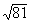The thought behind this is that we are looking for the square root of 81. This means that we are looking for a number that when we square it we get 81.  What do you think it is? Let's find out if you are right: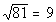Since 9 squared is 81, 9 is the square root of 81. Note that we are only interested in the principal root and since 81 is positive and there is not a sign in front of the radical, our answer is positive 9.  If there had been a negative in front of the radical our answer would have been -9.Answer/Discussion to 1b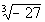The thought behind this is that we are looking for the cube root of -27. This means that we are looking for a number that when we cube it we get -27.  What do you think it is? Let's find out if you are right: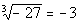Since -3 cubed is -27, -3 is the cube root of -27.Answer/Discussion to 1c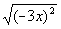Since it didn't say that x is positive, we have to assume that it can be either positive or negative.  And since the root number and exponent are equal we can use therule.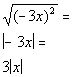Since the root number and the exponent inside are equal and are the even number 2, we need to put an absolute value around x for our answer.  The reason for the absolute value is that we do not know if x is positive or negative.  So if we put x as our answer and it was negative, it would not be a true statement.Answer/Discussion to 1d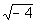Now we are looking for the square root of -4, which means we are looking for a number that when we square it we get -4. What do you think it is? Let's find out if you are right: Since there is no such real number that when we square it we get -4, then the answer is not a real number.Answer/Discussion to 2a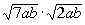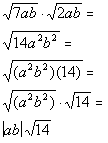*Use the prod. rule of radicals to rewrite

 Note that both radicals have an index number of 2, so we were able to put their product together under one radical keeping the 2 as its index number.  Since we cannot take the square root of 14 and 14 does not have any factors we can take the square root of, this is as simplified as it gets.Answer/Discussion to 2b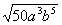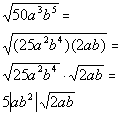*Use the prod. rule of radicals to rewrite

 In this example, we are using the product rule of radicals in reverse to help us simplify the cube root of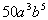.  When you simplify a radical, you want to take out as much as possible.  The factor ofthat we can take the square root of is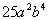.  We can writeas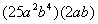and then use the product rule of radicals to separate the two numbers.  We can take the square root of, which is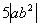, but we will have to leave the rest of it under the square root.Answer/Discussion to 3a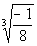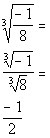*Use the  quotient rule of radicals to rewrite   *The cube root of -1 is -1 and the cube root of 8 is 2Answer/Discussion to 3b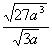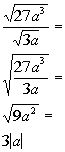*Use the  quotient rule of radicals to rewrite *Simplify the fraction *The square root of 9 a squared  is 3|a|Answer/Discussion to 4a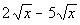Both radicals are as simplified as it gets.

 Step 2: Combine like radicals.

 Note how both radicals are the square root of x.  These two radicals are like radicals.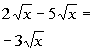*Combine like radicals: 2 - 5 = -3Answer/Discussion to 4b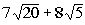The 20 in the first radical has a factor that we can take the square root of.  Can you think of what that factor is? Let's see what we get when we simplify the first radical: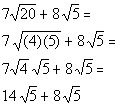*Rewrite 20 as (4)(5) *Use Prod. Rule of Radicals *Square root of 4 is 2

 Step 2: Combine like radicals.

 Note how both radicals are the square root of 5.  These two radicals are like radicals.*Combine like radicals: 14 + 8 = 22Answer/Discussion to 5aStep 1: Multiply numerator and denominator by a radical that will get rid of the radical in the denominator.

 Since we have a square root in the denominator,  we need to multiply by the square root of an expression that will give us a perfect square under the radical in the denominator. Square roots are nice to work with in this type of problem because if the radicand is not a perfect square to begin with, we just have to multiply it by itself and then we have a perfect square. So in this case we can accomplish this by multiplying top and bottom by the square root of 7:*Mult. num. and den. by sq. root of 7     *Den. now has a perfect square under sq. root

 AND

 Step 3: Simplify the fraction if needed.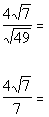*Sq. root of 49 is 7

 Be careful when you reduce a fraction like this.  It is real tempting to cancel the 7 which is on the outside of the radical with the 7 which is inside the radical on the last fraction.  You cannot do that unless they are both inside the same radical or both outside the radical.Answer/Discussion to 5b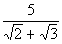Step 1: Find the conjugate of the denominator.

 In general the conjugate of a + b is a - b and vice versa. So what would the conjugate of our denominator be? It looks like the conjugate is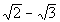.

 Step 2: Multiply the numerator and the denominator of the fraction by the conjugate found in Step 1.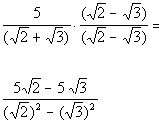*Mult. num. and den. by conjugate of den. *Use distributive prop. to multiply the numerators *In general, product of conjugates is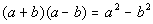AND

 Step 4: Simplify the fraction if needed.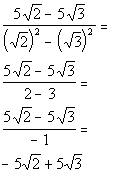*Square root of 2 squared is 2 and the square root of 3 squared is 3     *Divide BOTH terms of num. by -1

Last revised on Dec. 6, 2009 by Kim Seward.
All contents copyright (C) 2002 - 2010, WTAMU and Kim Seward. All rights reserved.